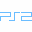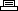Site SearchSponge Bob Squarepants: Battle For Bikini BottomPrinter Friendly Version

```1,000 shiny objects:
Pause game play, then hold L1 + L2 + R1 + R2 and quickly
press Square, Circle(2), Square(2), Circle(2), Square.
A sound will confirm correct code entry.

10 Golden Spatulas:
Pause game play, then hold L1 + L2 + R1 + R2 and quickly
press Circle, Square(2), Circle(2), Square(2), Circle. A
sound will confirm correct code entry.

Restore health:
Pause game play, then hold L1 + L2 + R1 + R2 and quickly
press Circle(4), Square, Circle, Square, Circle, Square(4).
A sound will confirm correct code entry.

Bubble bowl power-up:
Pause game play, then hold L1 + L2 + R1 + R2 and quickly
press Circle, Square, Circle, Square, Circle(2), Square(2).
A sound will confirm correct code entry.

Cruise bubble power-up:
Pause game play, then hold L1 + L2 + R1 + R2 and quickly
press Square, Circle, Square, Circle, Square(2), Circle(2).
A sound will confirm correct code entry.

All monsters in the gallery:
Pause game play, then hold L1 + L2 + R1 + R2 and quickly
press Circle, Square, Circle, Square(2), Circle, Square,
Circle. A sound will confirm correct code entry.

Pantless SpongeBob:
Pause game play, then hold L1 + L2 + R1 + R2 and quickly
press Circle(4), Square, Circle(2), Square, Circle, Square(2),
Circle. A sound will confirm correct code entry.

Giant plankton:
Pause game play, then hold L1 + L2 + R1 + R2 and quickly
press Square(4), Circle, Square, Circle, Square, Circle(4).
A sound will confirm correct code entry.

Small people (fishes):
Pause game play, then hold L1 + L2 + R1 + R2 and quickly
press Square(5), Circle, Square, Circle, Square, Circle,
Square, Circle. A sound will confirm correct code entry.

```